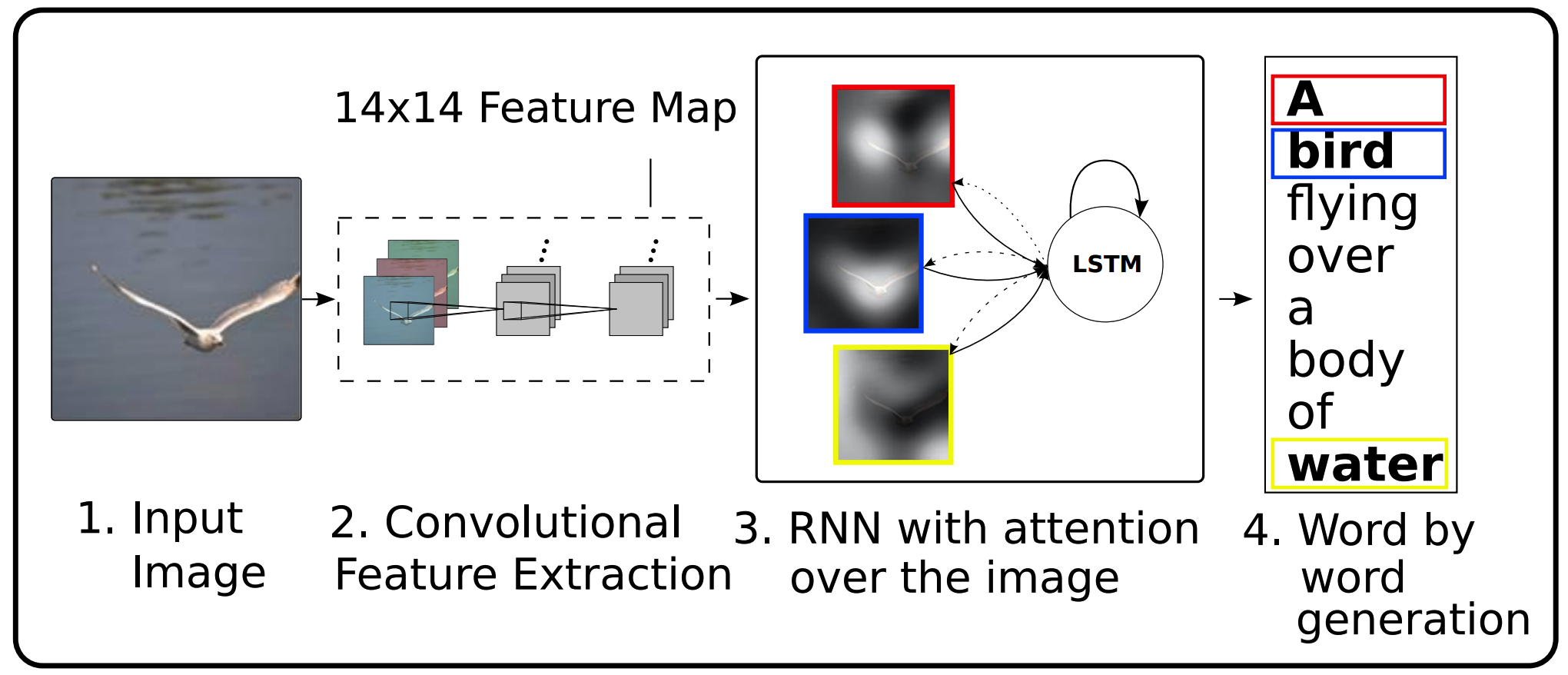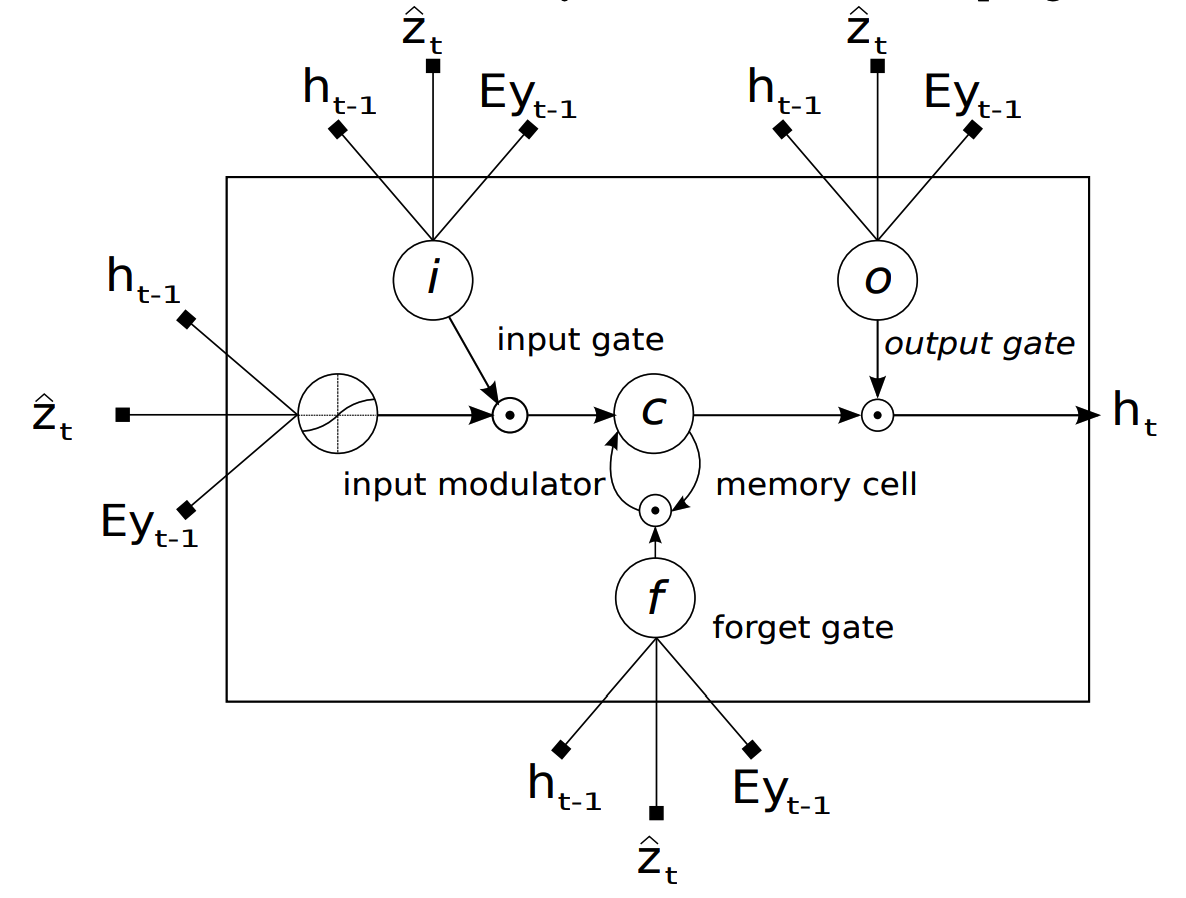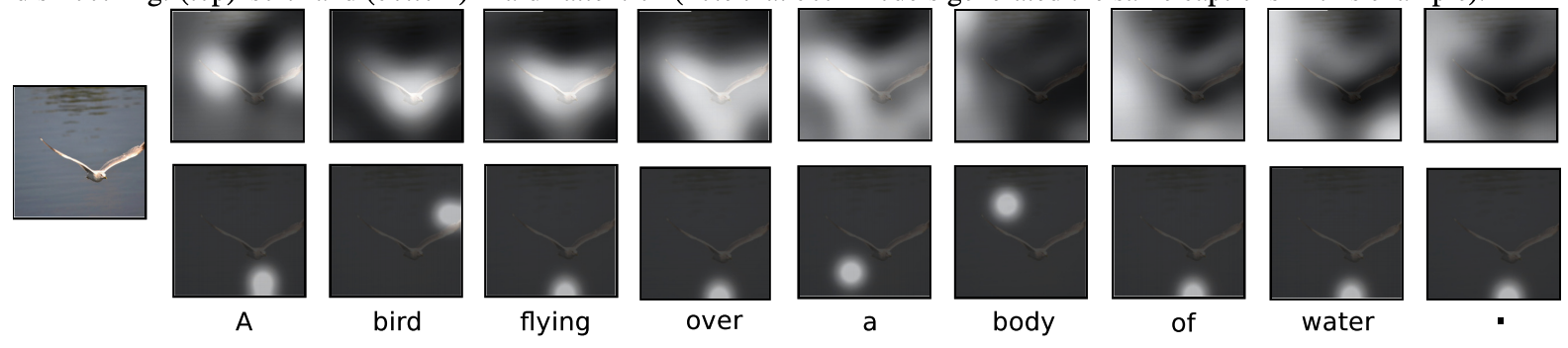# Show, Attend and Tell: Neural Image Caption Generation with Visual Attention

## WHY?

A caption of an image can be generated with attention based model by aligning a word to a part of image.

## WHAT?A convolution neural network extracts features from raw images resulting a series of feature vectors. In order to generate a series of words as a caption, LSTM with attention model is used. Generated feature vectors are used as annotation vectors for attention. Previous word, previous hidden vector and current context vector is concatenated and projected to the dimension of hidden vector to be used as inputs for gates. Deep output layer is used for output word probability distribution.e_{ti} = f_{att}(\mathbf{a_i}, \mathbf{h_{t-1}})\\
\alpha_{ti} = \frac{\exp(e_{ti})}{\sum_{k=1}^L \exp(e_{tk})}\\
\hat{z}_t = \phi(\{\mathbf{a}_i\}, \{\alpha_i\})\\
p(\mathbf{y}_\mathbf{a}, \mathbf{y}_1^{t-1}) \propto \exp(\mathbf{L}_o(\mathbf{E}\mathbf{y}_{t-1} + \mathbf{L}_h \mathbf{h}_t + \mathbf{L}_z\hat{\mathbf{z}}_t))

Weighting function of annoation vectors and a hidden vector(\phi$\phi$) can be varied. Stochastic hard attention treate the weights of attention as parameters of multinoulli distribution and sample a place of attention from the distribution. Variational lower bound on the marginal log-likelihood can be maximized by estimating gradients with REINFORCE algorithm. Deterministic soft attention take the expectation of context vector by weight summing annotation vectors. Doubly stochasic attention is used to encourage model to focus on the every part of the image by imposing regularization on the loss function.

L_d = -\log(P(\mathbf{y}|\mathbf{x})) + \lambda\sum^L_i(1 - \sum_t^C\alpha_{ti})^2

## So?Attention model was able to generate caption by sequentially focusing on the part of images.

## Critic

Attention of other words other than keywords were drifting around. There can be attention for relations since some words refer to the relations of the objects.

Powered by aiden